¡ì 5 The general idea of ​​stability theory

Stability theory studies how the solution changes when the right-hand side function of the differential equation changes with the initial conditions .

The concept of stability

[ Stability and instability of solutions ]   Let the system of differential equations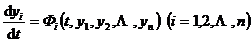The solution that satisfies the initial conditions is .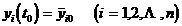If any given ¦Å > 0 , there is always a corresponding positive number ¦Ä = ¦Ä ( t 0  , ¦Å ) such that as long as the initial value satisfies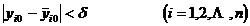The corresponding solution of this system of differential equations ( i = 1,2, ¡­ , n ) is satisfied for all t > t 0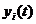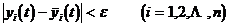The solution is said to be stable .Simply put , a solution is said to be stable if all solutions whose initial value is close to the initial value of a solution are always close to a solution when t > t 0 .

If for any given ¦Å > 0 , no matter how small ¦Ä > 0 is taken, there is always something satisfyingThe initial value of and ¦Ó > t 0 , its corresponding solution does not satisfy the condition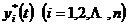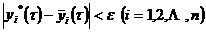The solution is said to be unstable .If stable, and the initial value satisfies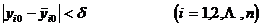All solutions for satisfiesis said to be asymptotically stable .[ Simplification of the problem ]   Given a system of equations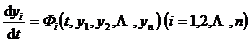In order to study the stability of its solution satisfying the initial conditions , the deviation of other solutions from it must be investigated .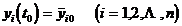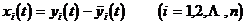The original system of equations becomes as follows: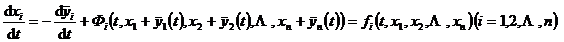(1)

The stability of the equations (1) is attributed to the stability of the zero solution x i ¡Ô 0 ( i =1,2, ¡­ , n ) of the system of equations (1) .The constant solution of any system of differential equations is often called its equilibrium point (singularity) . So the zero solution of (1 )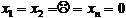is the balance point .

If any given ¦Å > 0 , there is always a corresponding positive number ¦Ä = ¦Ä ( t 0  , ¦Å ) such that as long as the initial value satisfies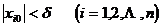The corresponding solution of (1 )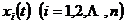is satisfied for all t > t 0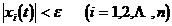Then the equilibrium point of (1) is said to be stable .If the condition is then metThe equilibrium point is said to be asymptotically stable .If no matter how small the positive number ¦Ä is chosen, for a predetermined positive number ¦Å , there is always a satisfactionThe initial value of and ¦Ó > t 0 , its corresponding solution does not satisfy the condition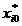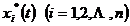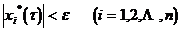Then the equilibrium point of (1) is said to be unstable .[ phase space ]   Given a system of equations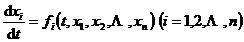The space of ( x 1 , x 2 , . _ _ _ _ _ _ _ _ _ _ A curve in phase space, called an orbit . In other cases, the solution curve is often called the integral curve .

The solution to the stability problem

[ Stability Problem of Equilibrium Points of Homogeneous Linear Differential Equations with Constant Coefficients ] For the sake of   simplicity , only the equations with two unknown functions are studied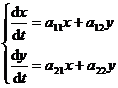where a 11 , a 12 , a 21 , and a 22 are all real numbers, and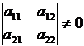from the characteristic equation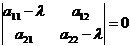Calculate the characteristic roots ¦Ë 1 , ¦Ë 2 , and substitute them into the following equations in turn: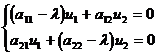Two sets of solutions 1 , 2 and .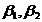At this time, the stability of the equilibrium point x ¡Ô 0, y ¡Ô 0 of the linear equation system can be discussed in the following cases .

1 ¡ã eigenroots are real numbers: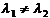The general solution is in the form of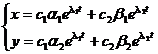where c 1 , c 2 are arbitrary constants .

( i )    1 < 0 , 2 < 0

The zero solution is asymptotically stable . The shape of the orbit is shown in Figure 13.1( a ) ( the arrow indicates the direction of t increasing, the same below) . This type of equilibrium point (0,0) is called a stable node .

( ii )   1 > 0 , 2 > 0

The zero solution is unstable . The orbital shape is shown in Figure 13.1( b ). This type of equilibrium point ( 0,0) is called an unstable node .

( iii ) 1 > 0 , 2 < 0

The zero solution is unstable . The orbital shape is shown in Figure 13.1( c ). This type of equilibrium point ( 0,0) is called a saddle point .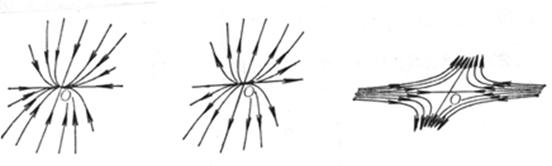( a ) ( b ) ( c )

Figure 13.1

2 ¡ã eigenroots are complex numbers:The general solution is in the form of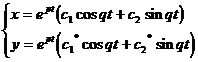where c 1 , c 2 are arbitrary constants, and c 1 *, c 2 * are linear combinations of c 1 , c 2 .

( i )    1 ,2 = p iq , p <0, q 0.

The zero solution is stable . The orbital shape is shown in Figure 13.2( a ). This type of equilibrium point ( 0,0) is called a stable focus .

( ii )   1 ,2 = p iq , p >0, q 0.

The zero solution is unstable . The orbital shape is shown in Figure 13.2( b ). This type of equilibrium point ( 0,0) is called an unstable focus .

( iii ) 1 , 2 = iq , q 0.

The zero solution is stable . The orbital shape is shown in Figure 13.2( c ). This type of equilibrium point ( 0,0) is called the center, and the center is stable .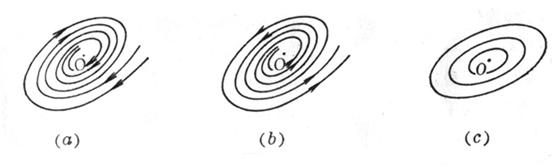Figure 13.2

The 3 ¡ã characteristic equation has multiple roots: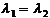The general solution is in the form of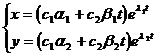( i )    1 = 2 < 0

The zero solution is asymptotically stable . The orbital shape is shown in Figure 13.3( a ). This type of equilibrium point ( 0,0) is called a stable degenerate node .

If the zero solution is a stable node, it is called a critical node . The shape of the orbit is shown in Figure 13.3 ( b ).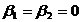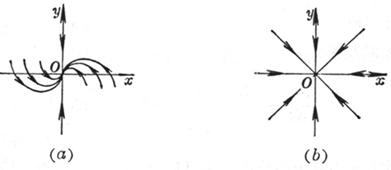Figure 13.3

( ii )   1 = 2 > 0

The zero solution is unstable . The orbital shapes are shown in Figure 13.3 ( a ) and ( b ), but the arrows are in opposite directions . This type of equilibrium point (0,0) is called the unstable degenerate node and the unstable critical node .

Combining the above situations, the following conclusions can be drawn: if the roots of the characteristic equation have negative real parts, then the zero solution is stable and asymptotically stable; if the characteristic equation has a root with a positive real part, then zero The solution is unstable .

This conclusion, for the general system of homogeneous linear differential equations with constant coefficients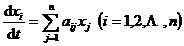is also established .

Theorem if the characteristic equation of a system of homogeneous linear differential equations with constant coefficients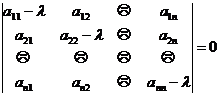A zero solution is asymptotically stable if all roots have negative real parts; a zero solution is unstable if at least one of all the roots of the characteristic equation has a positive real part .

[ Determining stability by first approximation ]   Consider the system of equations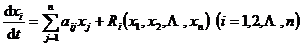where a ij ( i , j =1,2, ¡­ , n ) is a constant, and R i ( x 1 , x 2 , ¡­ , x n ) ( i = 1,2, ¡­ , n ) is a constant for lower than the second order .Its first-order approximate equation system is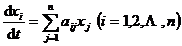Stability can be determined by studying various cases of the characteristic roots i ( i = 1,2, ¡­ , n ) of a system of first-order approximate equations . There are two basic theorems:

The first theorem If all the eigenvalues ​​of the first approximation equation system have negative real parts, then the zero solution of the original equation system is asymptotically stable .

The second theorem If at least one of the eigenvalues ​​of the first-order approximate equation system has a positive real part, then the zero solution of the original equation system is unstable .

These two theorems cover all stable cases (called noncritical cases) in which the zero solution of the original system of equations can be studied with a first-order approximation . As for at least one root with a zero real part, all other roots have negative In the critical case of the real part, the higher-order terms on the right-hand side of the equation system play an important role in the stability of the zero solution, so it is generally impossible to study the stability problem by the first-order approximation equation system .

[ Hurwitz Discriminant Method ]   It is a method to directly use some properties of the determinant formed by the coefficients of the characteristic equation to determine the stability of the zero solution of a system of linear differential equations with constant coefficients .

Let the characteristic equation of the system of linear differential equations with constant coefficients beThen the necessary and sufficient conditions for the zero solution of the system of constant coefficient linear differential equations to be asymptotically stable are: a 0 >0 , and all Hurwitz determinants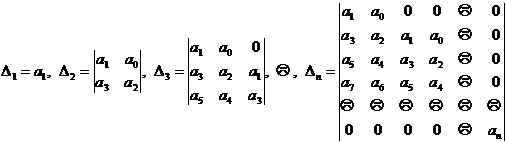are all positive (the final ¦¤ n > 0 can be replaced by the condition a n > 0 ) .

If ¦¤ n =0 , then since ¦¤ n = a n ¦¤ n -1 =0 , there must be an =0 or ¦¤ n -1 = 0 . If an = 0 , the characteristic equation has zero roots; if ¦¤ n -1 = 0 , then the characteristic equation has pure imaginary roots, in both cases the zero solution may or may not be stable .

The characteristic equation is the Hurwitz discriminant condition for quadratic, cubic and quartic (for the convenience of drawing, a 0 =1 is taken below ):

( i )    Characteristic equation:Hurwitz conditions are a 1 >0, a 2 >0

The stable region is shown in Figure 13.4( a ).

( ii )   Characteristic equation: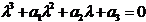The Hurwitz condition is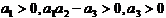The stable region is shown in Figure 13.4( b ).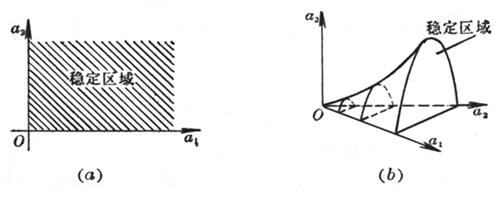Figure 13.4

( iii ) Characteristic equation:The Hurwitz condition is .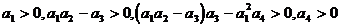[ Lyapunov's second method (direct method) ] to   study systems of differential equations(1)

There is a more general method for the stability of the equilibrium point of , the so-called Lyapunov method .

1 ¡ã Lyapunov stability theorem For the system of equations (1) , if one can find a differentiable function V ( x 1 , x 2 , ¡­ , x n ) (called the Lyapunov function ) that satisfies the following conditions in the neighborhood of the origin ):

( i )    V ( x 1 , x 2 , ¡­ , x n ) ¡Ý 0 (or ¡Ü 0 ), and only when x i =0 ( i =1,2, ¡­ , n ) , V =0 .

( ii )   When t ¡Ý t 0 , the full derivative of V along the integral curve of the system of equations ( 1)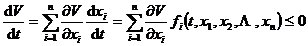( or )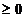Then the equilibrium point x i =0 ( i =1,2, ... , n ) of the system of equations (1 ) is stable .

2 ¡ã Lyapunov Asymptotic Stability Theorem For the system of equations (1) , if one can find a differentiable function V ( x 1 , x 2 , ¡­ , x n ) in the neighborhood of the origin that satisfies the following conditions (called Lyapunov Nov function):

( i )    V ( x 1 , x 2 , ¡­ , x n ) ¡Ý 0 (or ¡Ü 0 ), and only when x i =0 ( i =1,2, ¡­ , n ) , V =0 .

( ii )   The total derivative along the integral curve of the system of equations ( 1)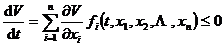( or )And outside a suitably small delta neighborhood of the origin (ie ), when t ¡Ý t 0 , (or ) then the equilibrium point x i =0 ( i =1,2, ¡­ , n ) of the system of equations (1 ) is asymptotically nearly stable .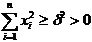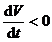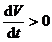Case Study of Differential Equations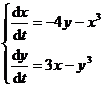Stability at the equilibrium point x = 0, y = 0 .

The characteristic equation of the first-order approximation equation for solving this system of equations has two pure imaginary roots, so it is a critical case and cannot be studied by the first-order approximation method . Now use the Lyapunov method . Takebecause

( i )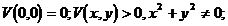( ii )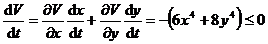For any ¦Ä > 0 , when t > t 0 , . Therefore, the equilibrium point (0,0) is asymptotically stable .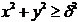3. Limit cycle (or limit cycle)

Only the case of n = 2 is discussed here .

[ periodic solution ]   equation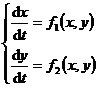A periodic solution with period T is a solution that satisfies x ( t + T ) = x ( t ) and y ( t+T ) = y ( t ) . The orbit corresponding to the periodic solution is a closed curve . Conversely, a closed orbit corresponds to the periodic solution .

[ Limit cycle ]   The isolated periodic solution is called the limit cycle of the equation . In a complete way , it is: let x=x ( t ), y=y ( t ) be the periodic solution of the equation, and K is the solution drawn on the phase plane The closed curve of . If there is a positive number ¦Ñ , such that for any point ¦Æ on the phase plane whose distance from K is less than ¦Ñ , the solution of the equation through the point ¦Æ is not periodic, then x=x ( t ) , y=y ( t ) (that is, the closed orbit K ) is an isolated periodic solution, or a limit cycle .

example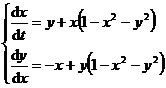Do coordinate transformation: x = r cos , y = r sin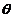, the equations are transformed into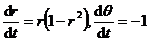The general solution is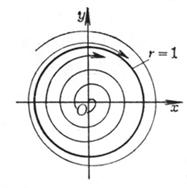Figure 13.5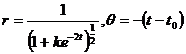Where k , t 0 are arbitrary . Take t 0 =0 , then the solution of the system of equations isWhen k= 0 , it is a circle x 2 + y 2 =1 ; when k=c 2 ( c> 0) , it is a spiral, when t - , it tends to the origin, and when t , it is approached from the inner spiral Circumference x 2 + y 2 =1 ; when k= ( c> 0) , the orbit is a curve, when t log c +0 , it tends to infinity, and when t , it circles from the outside to approximate the circumference x 2 + y 2 =1.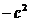The track distribution is shown in Figure 13.5.

Then the circumference x 2 + y 2 =1 is the only limit cycle of the system of equations . (0,0) is the only singular point .

[ Limit cycle existence theorem ]   For the system of equations1 ¡ã There are two simple closed curves C 1 and C 2 on the xy plane , C 2 is inside C 1 , and the following two conditions are satisfied:

( i )    The vector field of a point on C1 points from the outside of C1 to the inside, and the vector field of a point on C2 points from the inside of C2 to the outside ;

( ii )   There is no singularity in the system of equations in the annular region enclosed by C 1 and C 2 ;

Then in the annular region enclosed by C 1 and C 2 , there must be a stable limit cycle (called the Poincare - Bendikesen theorem) .

2 ¡ã If in a simple connected region G , the sign is constant, and it is not equal to zero in any subregion D ( G ) , then in G , the system of equations does not have any closed orbits . ©¡